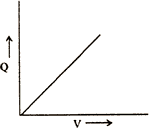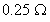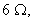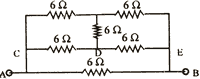Visitors Online: 77 | Sunday 18th August 2019
 CBSE Guess > Papers > Question Papers > Class XII > 2003 > Physics > Outside Delhi Set -II PHYSICS—2003 (Set II—Outside Delhi)
Note: Except for the following questions, all the remaining questions have already been asked in Set I . Q. 1. Write the S.L unit of (i) magnetic pole strength, (ii) magnetic dipole moment of a bar magnet. Q. 2. A lens of glass is immersed in water. What will be its effect on the power of the lens? Q. 3. Sketch a graph to show how the charge 'Q' acquired by a capacitor of capacitance 'C' varies with increase in potential difference between its plates.Q. 5. The given graph shows the variation of resistance of mercury in the temperature range 0 < T < 4 K. Name the phenomenon shown by the graph.Q. 6. With the help of diagrams, differentiate between (i) pulse-amplitude modulation (PAM) and (ii) pulse-position modulation (PPM). Q. 7. How is an n-type semiconductor formed? Name the major charge carriers in it. Draw the energy band diagram of an n-type semiconductor. Q. 11. Two similarly and equally charged identical metal spheres A and B repel each other with a force of 2 x 10 -5 N. A third identical uncharged sphere C is touched with A and then placed at the mid-point between A and B. Calculate the net electric force on C. Q. 14. Draw a labelled circuit diagram of a common base amplifier using a n-p-n transistor. Name the purpose for which common base transistor amplifier is preferred over common emitter transistor amplifier. Q. 16. Define critical angle with reference to total internal reflection. Calculate the critical angle for glass - air surface, if a ray of light which is incident in air on the glass surface is deviated through 150, when angle of incidence is 450. Q. 19.town situated 60 km away from a power plant generating power at 440 V, requires 400 kW of electric power at 220 V. The resistance of the two wire line carrying power isper km. The town gets power from the line through a 3000 - 220 V step down transformer at a substation in the town. (i) Find the line power losses in the form of heat. (ii) How much power must the plant supply, assuming there is negligible power loss due to leakage? Q. 23. Six resistors, each of valueare joined together in a circuit as shown in the figure. Calculate equivalent resistance across the points A and B. If a cell of emf 3 V is connected across AB, compute the current through the arms AB and DF of the circuit.Physics 2003 Question Papers Class XII Delhi Outside Delhi Compartment Delhi Compartment Delhi Set I Set I Set I Set I Set II Set II Set II Set II CBSE 2003 Question Papers Class XII